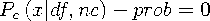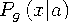Functions and CALL Routines

# CNONCT Function

Returns the noncentrality parameter from a chi-square distribution.
 Category: Mathematical

## Syntax

 CNONCT(x,df,prob)

### Arguments

x

is a numeric random variable.

 Range: x0
df

is a numeric degrees of freedom parameter.

 Range: df > 0
prob

is a probability.

 Range: 0 < prob < 1

The CNONCT function returns the nonnegative noncentrality parameter from a noncentral chi-square distribution whose parameters are x, df, and nc. If prob is greater than the probability from the central chi-square distribution with the parameters x and df, a root to this problem does not exist. In this case a missing value is returned. A Newton-type algorithm is used to find a nonnegative root nc of the equationwherewhereis the probability from the gamma distribution given byIf the algorithm fails to converge to a fixed point, a missing value is returned.

```data work;
x=2;
df=4;
do nc=1 to 3 by .5;
prob=probchi(x,df,nc);
ncc=cnonct(x,df,prob);
output;
end;
run;
proc print;
run;```

```            OBS    x    df     nc      prob     ncc

1     2     4    1.0    0.18611    1.0
2     2     4    1.5    0.15592    1.5
3     2     4    2.0    0.13048    2.0
4     2     4    2.5    0.10907    2.5
5     2     4    3.0    0.09109    3.0```Previous Page | Next Page | Top of Page